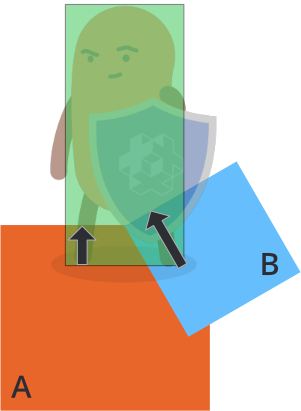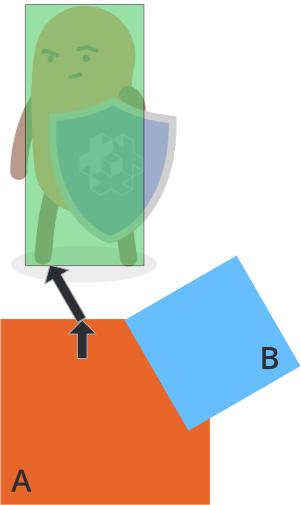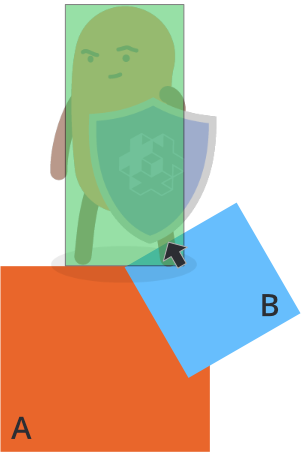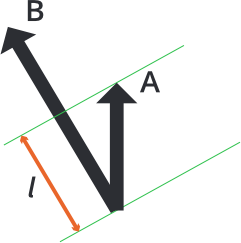# 處理动画碰撞

function on_message(self, message_id, message, sender)
-- 处理碰撞
if message_id == hash("contact_point_response") then
local newpos = go.get_position() + message.normal * message.distance
go.set_position(newpos)
end
end• 根据 A 的穿透距离把角色向上移 (黑色箭头)
• 根据 B 的穿透距离把角色向左上移 (黑色箭头)$l = vmath.project(A, B) \times vmath.length(B)$

1. 把当前矫正向量映射到碰撞穿透向量上.
2. 计算穿透向量的补偿 (按照上述公式).
3. 依照补偿向量移动对象.
4. 把补偿向量累加到矫正向量中.

function init(self)
-- 校正向量
self.correction = vmath.vector3()
end

function update(self, dt)
-- 重置矫正向量
self.correction = vmath.vector3()
end

function on_message(self, message_id, message, sender)
-- 处理碰撞
if message_id == hash("contact_point_response") then
-- 获取位移计算所需数据.
-- 当前帧可能有多个碰撞点需要处理,
-- 通过累积矫正向量,
-- 达到正确计算位移的目的:
if message.distance > 0 then
-- 第一步, 把矫正向量投射到
-- 穿透向量上.
local proj = vmath.project(self.correction, message.normal * message.distance)
if proj < 1 then
-- 没有过冲的才需要补偿.
local comp = (message.distance - message.distance * proj) * message.normal
-- 应用补偿向量.
go.set_position(go.get_position() + comp)
-- 积累矫正向量.
self.correction = self.correction + comp
end
end
end
end


Did you spot an error or do you have a suggestion? Please let us know on GitHub!